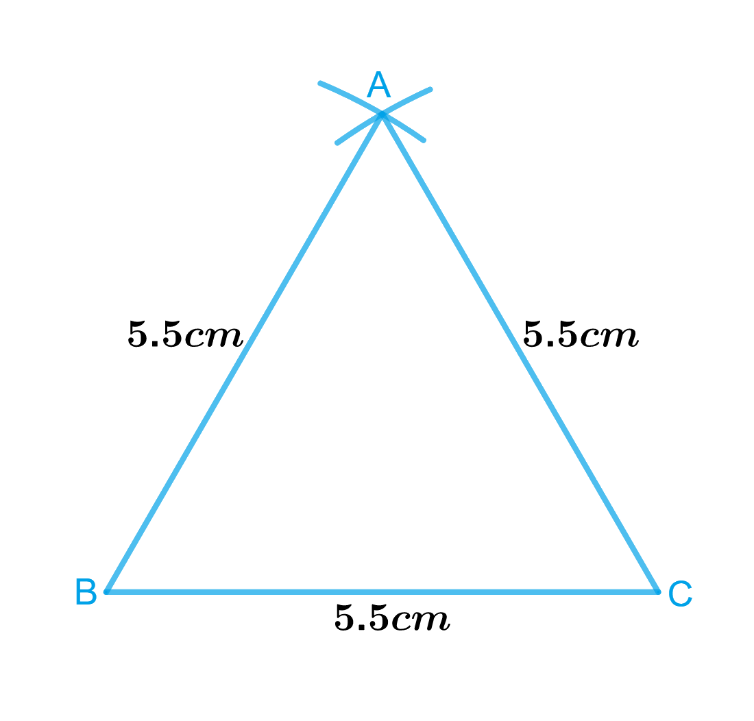# Ex.10.2 Q2 Practical Geometry Solution- NCERT Maths Class 7

Go back to  'Ex.10.2'

## Question

Construct an equilateral triangle of side $$5.5\,\rm{cm}$$.

Video Solution
Practical Geometry
Ex 10.2 | Question 2

## Text Solution

What is known?

Lengths of sides of an equilateral triangle are $$5.5\,\rm{cm}$$ each.

To construct:

An equilateral triangle of side $$5.5\,\rm{cm}$$.

Reasoning:

To construct an equilateral triangle of side $$5.5\,\rm{cm}$$. Follow the steps given below

Steps:Steps of construction :

1. Draw a line segment $$BC$$ of length $$5.5\,\rm{cm}$$.
2. From $$B$$, point $$A$$ is at a distance of $$5.5\,\rm{cm}$$.So, with $$B$$ as centre, draw an arc of radius $$5.5\,\rm{cm}$$ (Now $$A$$ will be somewhere on this arc. Our job is to find where exactly $$A$$ is).
3. From $$C$$, point $$A$$ is at a distance of $$5.5\,\rm{cm}$$.So, with $$C$$ as centre, draw an arc of radius $$5.5\,\rm{cm}$$ (Now $$A$$ will be somewhere on this arc, we have to fix it).
4. $$A$$ has to be on both the arcs drawn. So, it is the point of intersection of arcs. Mark the point of intersection of arcs as $$A$$ join $$AB$$ and $$AC$$.

Thus, $$ABC$$ is the required triangle.

Learn from the best math teachers and top your exams

• Live one on one classroom and doubt clearing
• Practice worksheets in and after class for conceptual clarity
• Personalized curriculum to keep up with school